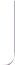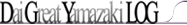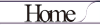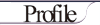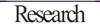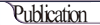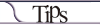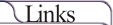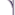CMB
BBNCMB
I. Primordial magnetic fields and CMB

Primordial magnetic fields (PMFs), which were generated in the early universe before recombination, affect the motion of plasma and then the cosmic microwave background (CMB) and the matter power spectrum (MPS). We consider constraints on PMFs with a characteristic correlation length from the observations of the anisotropies of CMB (WMAP, QUAD, ACT, SPT, and ACBAR) and MPS. The spectrum of PMFs is modeled with multi-lognormal distributions (MLND), rather than power-law distribution, and we derive constraints on the strength |B ( k )| at each wavenumber k along with the standard cosmological parameters in the flat Universe and the foreground sources. We obtain upper bounds on the field strengths at k = 10-1, -2, -4. -5 Mpc-1 as 4.7 nG, 2.1 nG, 5.3 nG and 10.9 nG (2σ C.L.) respectively, while the field strength at k = 10-3 Mpc-1 turns out to have a finite value as
|B ( k =10-3 Mpc-1 )| = 6.2 ± 1.3 nG (1σ C.L.).
This finite value is attributed to the finite values of BB mode data at l > 300 obtained from QUAD experiment. If we do not include the BB mode data, we obtain only the upper bound on |B ( k =10-3 Mpc-1 )|.
Please visit here (details in Japanese) for more detail.
[arXiv:1311.2584]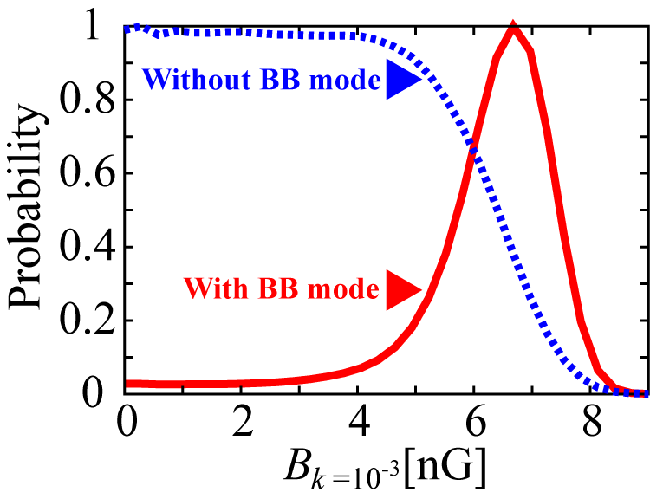Fig. 1; Probability distributions of the PMF strengths at k = 10-3 Mpc-1 from the CMB and the MPS data with the BB mode (solid) and without (dotted).BBN
Effects of primordial magnetic field on BBN
Big bang nucleosynthesis (BBN) is affected by the energy density of a primordial magnetic field (PMF). For an easy derivation of constraints on models for PMF generations, we assume a PMF with a power law (PL) distribution in wave number defined with a field strength, a PL index, and maximum and minimum scales at a generation epoch. We then show a relation between PL-PMF parameters and the scale invariant (SI) strength of PMF for the first time. We perform a BBN calculation including PMF effects, and show abundances as a function of baryon to photon ratio $\eta$. The SI-PMF strength is constrained from observational constraints on 4He and D. The minimum abundance of 7Li/H as a function of $\eta$ slightly moves to a higher 7Li/H value at a larger $\eta$ value when a PMF exists during BBN. We then discuss degeneracies between the PL-PMF parameters in the PMF effect. We also assume a general case in which both the existence and the dissipation of PMF are possible. It is then found that an upper limit on the SI-PMF strength can be derived from a constraint on 4He abundance, and that a lower limit on the allowed 7Li abundance is significantly higher than those observed in metal-poor stars.
We investigated constraints on parameters of the PL-PMF from the light elements abundance up to Li produced in BBN. We showed respective and combined constraints on the parameters. As a result, we obtained upper limits on the SI-PMF strength, i.e.,
BSI < 1.45 - 1.95 × 10-6 G
from abundances of 4He and D for the 2 $\sigma$ region of $\eta$ values by the WMAP 7yr data.
Please visit here (details in Japanese) for more detail.
[arXiv:1212.2968]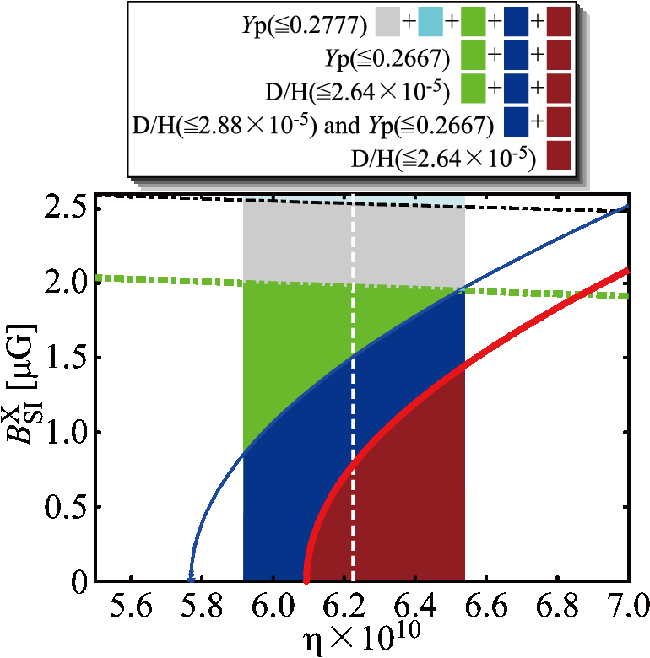Fig. 1; Constraints on the PMF strength by BBN. The vertical painted band is the limit on the baryon to photon ratio from WMAP 7yr data. The white dashed line indicates the best value. Painted regions in this figure are as indicated in the legend box at the top.